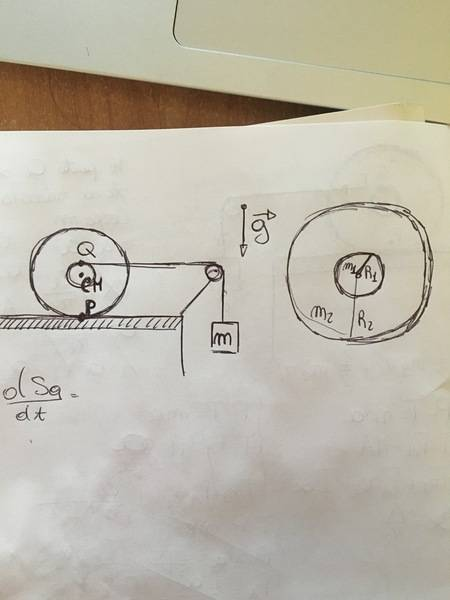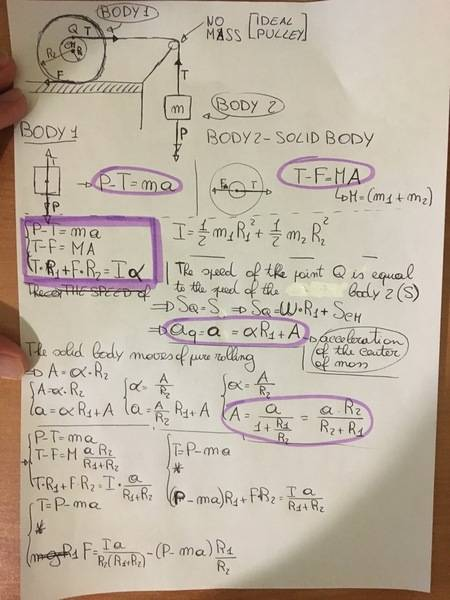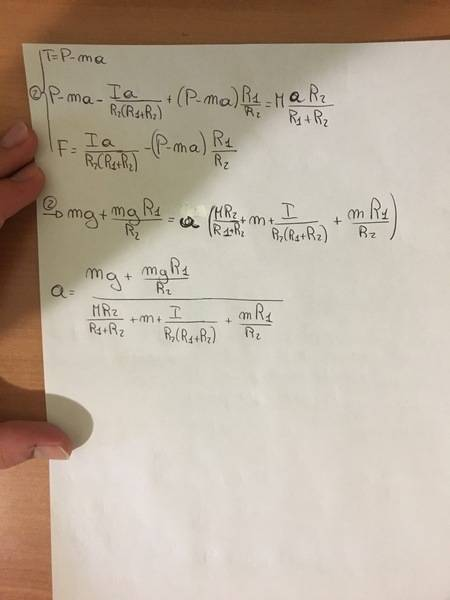# Problem about a rigid body pulled by a mass

## Homework Statement

A solid body consisting in two concentric disks of masses m1, m2 and radius r1<r2 is linked through a ideal rope to a mass m which falls down with a certain acceleration. The solid body moves of pure rolling.
Find the acceleration a of the mass.## Homework Equations

P-T=m*a
T-F=(m1+m2)*A

I=1/2*m1*r1^2+1/2*m2*r2^2
T*r1+F*r2=I*alpha

SQ=w*r1+Scm or Sq=w*r1
a=aQ=alpha*r1+A or a=aQ=alpha*r1
A=alpha*r2

## The Attempt at a Solution

The mass m is subject to its weight force P=m*g and the tension T of the rope so:

P-T=m*a
T-F=(m1+m2)*a

On the solid body the tension and the grip of the floor (F) act:
T-F=(m1+m2)*A
I chose the center of mass (cm) as the pole of momentum of forces:
T*r1+F*r2=I*alpha
I=1/2*m1*r1^2+1/2*m2*r2^2
now I tried to find a relation between a and alpha: the acceleration and the speed of the piont Q has to be equal to the speed and the acceleration of the mass because the rope can't extend. So SQ=w*r1+Scm and a=aQ=alpha*r1+A (indeed I don't know if SQ=w*r1+Scm or simply SQ=w*r1 in fact, in the solution of the problem I found that SQ=w*(r1+r2) but it has chosen the point P as pole for the momentum on the forces which ita speed is istant by istant naught). Since the body rolls without slither, the acceleration A of the center of mass and alpha must satisfy the condition A=alpha*r2.
I solved the sistem of equations (I tried both the equations for the speed) but in any case my solution was wrong. I hope you can help me.
Thanks a lot

#### Attachments

collinsmark
Homework Helper
Gold Member
Hello @MathError, welcome to Physics Forums!I haven't worked through the solution yet myself for these reasons: (A) there are some ambiguities in the problem statement and (B) once you get the ambiguities addressed you need to show more of your work.

Ambiguity (i):

Does disk 2 have a hole in the center allowing disk 1 to slide through (before they are attached together)? Or, is disk 2 completely solid, with disk 1 pasted on at its side?

Although there might be special cases where it doesn't matter (given the densities of the disks, etc.), the distinction does matter for the more general case.

For what it's worth, your initial attempt treated disk 1 being pasted onto the side of disk 2 (i.e., both are solid disks without holes). I just want to make sure that you are using the correct assumption in your approach.

Ambiguity (ii):

Are we supposed to find the linear acceleration a of the hanging mass or the cm of the rolling mass? It's not quite clear which mass is being discussed where.

The same is true in your attempted solution. Your attempt has the variable "A" in it in a few places, but it's not always clear to me which acceleration it refers to.

It would be helpful to everyone involved if you could define your variables more clearly.

It's not sufficient to say that you solved the system of equations and got the wrong answer. You need to show us exactly how you set up your system of equations, show us your solution, and tell us how you know that your solution was wrong. [Edit: or if you are stuck, show us exactly where you are stuck.]

## Homework Statement

A solid body consisting in two concentric disks of masses m1, m2 and radius r1<r2 is linked through a ideal rope to a mass m which falls down with a certain acceleration. The solid body moves of pure rolling.
Find the acceleration a of the mass.
View attachment 228745

## Homework Equations

P-T=m*a
T-F=(m1+m2)*A

I=1/2*m1*r1^2+1/2*m2*r2^2
T*r1+F*r2=I*alpha

SQ=w*r1+Scm or Sq=w*r1
a=aQ=alpha*r1+A or a=aQ=alpha*r1
A=alpha*r2

## The Attempt at a Solution

The mass m is subject to its weight force P=m*g and the tension T of the rope so:

P-T=m*a
T-F=(m1+m2)*a

On the solid body the tension and the grip of the floor (F) act:
T-F=(m1+m2)*A
I chose the center of mass (cm) as the pole of momentum of forces:
T*r1+F*r2=I*alpha
I=1/2*m1*r1^2+1/2*m2*r2^2
now I tried to find a relation between a and alpha: the acceleration and the speed of the piont Q has to be equal to the speed and the acceleration of the mass because the rope can't extend. So SQ=w*r1+Scm and a=aQ=alpha*r1+A (indeed I don't know if SQ=w*r1+Scm or simply SQ=w*r1 in fact, in the solution of the problem I found that SQ=w*(r1+r2) but it has chosen the point P as pole for the momentum on the forces which ita speed is istant by istant naught). Since the body rolls without slither, the acceleration A of the center of mass and alpha must satisfy the condition A=alpha*r2.
I solved the sistem of equations (I tried both the equations for the speed) but in any case my solution was wrong. I hope you can help me.
Thanks a lot

haruspex
Homework Helper
Gold Member
SQ=w*(r1+r2)
Yes.
it has chosen the point P as pole for the momentum on the forces which ita speed is istant by istant naught).
That is as clear as mud.
acceleration A of the center of mass and alpha must satisfy the condition A=alpha*r2.
Yes.
there are some ambiguities in the problem statement
I think it is fairly clear that the disks are supposed to be coaxial, not concentric as stated.

collinsmark
Homework Helper
Gold Member
I think it is fairly clear that the disks are supposed to be coaxial, not concentric as stated.
Well, changing from "concentric" to "coaxial" doesn't clear up any of the ambiguity in my eyes. A small disk passing through the hollow center of a larger disk are both coaxial, as is a smaller disk pasted to the sides of a solid, larger disk.

But I concede the that the "solid body" term in "A solid body consisting in two concentric disks..." loosely implies that that both disks are made of the same material, i.e, both disks have the same density; that's the special case where the configuration doesn't matter.

So if we assume that both disks together comprise a single piece of homogeneous material, then yes, none of this matters, and I withdraw my "Ambiguity (i)" comment.[Edit: On second thought, even that doesn't clear up all the ambiguity. See below.]

[Edit: although in a practical sense, it does have an impact on how the mass of each "disk" is calculated and what that means geometrically. To correctly solve this problem, it's important to know whether the stuff in very center of disk 2 actually contributes to m2 or whether that's really a part of m1. So there's still a bit of ambiguity left.]

Last edited:
haruspex
Homework Helper
Gold Member
A small disk passing through the hollow center of a larger disk are both coaxial
That is true, but it would be far more natural to refer to that arrangement as concentric, so use of coaxial would strongly suggest each is a complete disc and they are side by side.
And they are referred to as both being discs, not one disc and one annulus.

•collinsmark
collinsmark
Homework Helper
Gold Member
That is true, but it would be far more natural to refer to that arrangement as concentric, so use of coaxial would strongly suggest each is a complete disc and they are side by side.
And they are referred to as both being discs, not one disc and one annulus.
I agree, but that's where the ambiguity is. They are both referred to as "disks" (rather than one being an "annulus" or "disk with a hole in it") and yet the term "concentric" was chosen rather than "coaxial." Either they're concentric disk+annulus, or they're coaxial disks. But it doesn't make sense to have concentric disks of homogeneous material (i.e., that leads to two solid objects occupying the same space at the same time).

None of this would matter if it were stated that the "solid body" was made of homogeneous material, and instead of m1 and m2, the cylindrical heights h1 and h2 were given instead. That way one could calculate m1 and m2 however one preferred. But since m1 and m2 were given, the student must know of how the creator of the problem defined the geometry of these masses.

Hello, thanks for the answers, I'm sorry for the abiguities in the text so I'll try to be more clear.
The two disks are completely solid in fact in the section "relevant equations" I wrote the equation for the moment of inertia which is I=1/2*m1*r1^2+1/2*m2*r2^2.
The variable a rapresents the acceleration of the mass which is falling down while A is the acceleration of the center of mass of the solid body and alpha is the angular acceleration of the solid body.
I didn't write all my solution beacause I thought it would have made the text even harder to understand. So I reported all the equations I worked out and I tried to explain my doubts finding the relation betwenn a,A and alpha, in fact the solution writes SQ=w*(r1+r2), so a=alpha*(r1+r2), A=alpha*r2, A=a*r2/(r1+r2). However it assumed the point P, as pole for the momentum of forces which has zero speed. Instead I tried to solve the problem from the center of mass but I think I made some mistakes calculating the momentum of forces or finding the relation between a,A, and alpha as I wrote in this section of the text.
T*r1+F*r2=I*alpha
I=1/2*m1*r1^2+1/2*m2*r2^2
now I tried to find a relation between a and alpha: the acceleration and the speed of the piont Q has to be equal to the speed and the acceleration of the mass because the rope can't extend. So SQ=w*r1+Scm and a=aQ=alpha*r1+A (indeed I don't know if SQ=w*r1+Scm or simply SQ=w*r1 in fact, in the solution of the problem I found that SQ=w*(r1+r2) but it has chosen the point P as pole for the momentum on the forces which ita speed is istant by istant naught). Since the body rolls without slither, the acceleration A of the center of mass and alpha must satisfy the condition A=alpha*r2

haruspex
Homework Helper
Gold Member
I think I made some mistakes calculating the momentum of forces or finding the relation between a,A, and alpha
Maybe, but I could not identify any in your post, so you will need to write out all your steps.#### Attachments

haruspex
Homework Helper
Gold Member
I get the same answer using a more direct method (moments about P, e.g.).
Why do you think it is wrong? It can be written a little more neatly.

I'm sorry, I checked one more time the results, fixing the values, and they agree, so I made some algebric mistaskes.
However I want to make sure I understand the problem: if I didn't add the speed of the CM in the equation SQ=w*r1+Scm, wouldn't I consider the traslation of the CM? And why, using P as pole for the momentum, didn't it add CM's speed? (Maybe because it has no speed but I'm not sure)

haruspex
Homework Helper
Gold Member
if I didn't add the speed of the CM in the equation SQ=w*r1+Scm,
Why would you not add it?
wouldn't I consider the traslation of the CM?
I don't understand what you are suggesting in terms of equations.
why, using P as pole for the momentum, didn't it add CM's speed?
In general, you can consider the motion of a rigid body as the sum of a translation of and a rotation about a point fixed within the body. The two commonest choices are the mass centre and the instantaneous centre of rotation. If you choose the second then there is, by definition, no translation, and the entire motion can be expressed as a rotation about that point.
For a rolling disc or sphere, the point of contact is the instantaneous centre of rotation.

For what it is worth, this is my solution:
We can eliminate the pulley, making the string go horizontally all the way to the mass, with mg acting horizontally on it. Now we can express everything in terms of torques and moments of inertia about P:
##mg(r_1+r_2)=\alpha(m(r_1+r_2)^2+Mr_2^2+I)##

Last edited:
ok, thanks a lot, I think I understand the problem